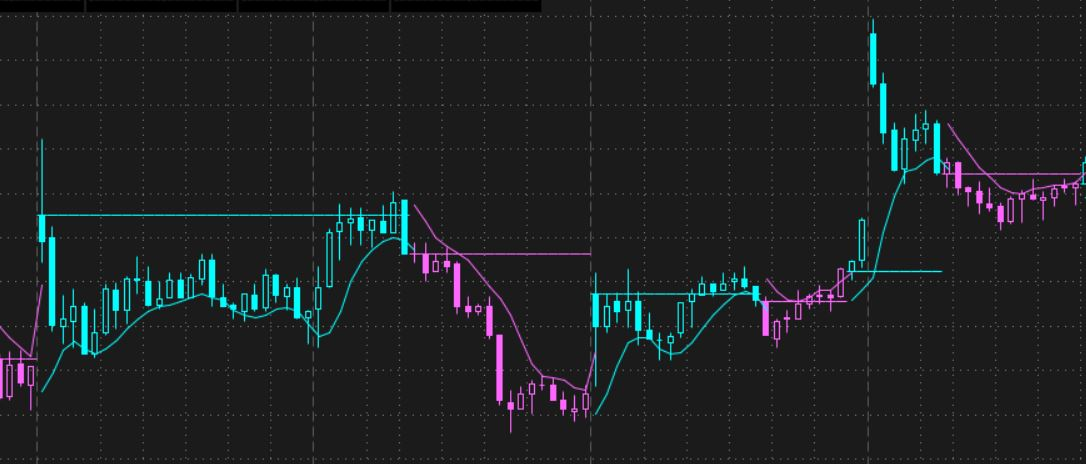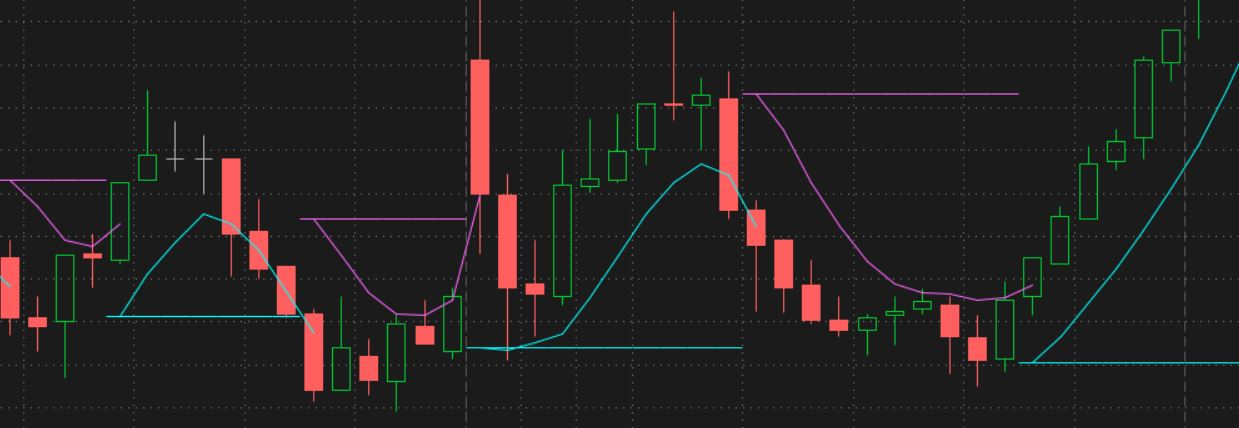# Need help plotting horizontal lines in place of coloring candles.

#### METAL

##### Active member
Plus
I would like to have the buy stop and sell stop plot a horizontal line intead of painting candles. Maybe have input option that give the option to use one or the other or both.
Code:
``````#
# TD Ameritrade IP Company, Inc. (c) 2020-2022
#

input length = 3;

def HAclose   =  (open + high + low + close) / 4;
def HAopen    = CompoundValue( 1, ( HAopen + HAclose ) / 2, HAclose );
def HAhigh    = Max( high, Max( HAopen, HAclose ) );
def HAlow     = Min( low, Min( HAopen, HAclose ) );

def maHigh = Average(HAhigh, length);
def maLow = Average(HAlow, length);
def state = {default init, short, long};
if (HAclose > maHigh) {
state = state.long;
} else if (HAclose < maLow) {
state = state.short;
} else {
state = state;
}

plot BuyStop = if state == state.short or state != state then maHigh else Double.NaN;
plot SellStop = if state == state.long or state != state then maLow else Double.NaN;

SellStop.SetDefaultColor(GetColor(1));

AssignPriceColor(if State == State.Long then SellStop.takevalueColor() else BuyStop.takevalueColor());

SellStop.SetDefaultColor(GetColor(1));``````

Solution
Thank You. This is close but I would like for the Horizontal stop lines to start where the original pink and cyan lines started. It looks as though they start at the top/bottom of previous candle? I looked in the code and I could not determine how this was coded. I think it is the  but you added that to your portion. Is there a way to make the stops customizable? some way to add a value in order to achieve the offset one would like.

sorry about that first version, i rushed it and didn't use the correct price level.

here is an updated version, that has the horizontal lines starting where the curve lines start.

Code:
``# trailstop_ha_signals_01...``
I would like to have the buy stop and sell stop plot a horizontal line intead of painting candles. Maybe have input option that give the option to use one or the other or both.
Code:
``````#
# TD Ameritrade IP Company, Inc. (c) 2020-2022
#

input length = 3;

def HAclose   =  (open + high + low + close) / 4;
def HAopen    = CompoundValue( 1, ( HAopen + HAclose ) / 2, HAclose );
def HAhigh    = Max( high, Max( HAopen, HAclose ) );
def HAlow     = Min( low, Min( HAopen, HAclose ) );

def maHigh = Average(HAhigh, length);
def maLow = Average(HAlow, length);
def state = {default init, short, long};
if (HAclose > maHigh) {
state = state.long;
} else if (HAclose < maLow) {
state = state.short;
} else {
state = state;
}

plot BuyStop = if state == state.short or state != state then maHigh else Double.NaN;
plot SellStop = if state == state.long or state != state then maLow else Double.NaN;

SellStop.SetDefaultColor(GetColor(1));

AssignPriceColor(if State == State.Long then SellStop.takevalueColor() else BuyStop.takevalueColor());

SellStop.SetDefaultColor(GetColor(1));``````

EDIT - this version doesn't draw the horizontal line at the desired HA price level

see post #4 below

--------------------------------

you didn't specify when and where you wanted horizontal lines, so i guessed, to start when the stop lines change colors.
i set the default price as the bar open as the start of the line. can be changed.
can turn bar color on/off.

Code:
``````# horz_lines_at_signals

#Need help plotting horizontal lines in place of coloring candles.

def na = double.nan;
def bn = barnumber();
# TD Ameritrade IP Company, Inc. (c) 2020-2022

input length = 3;

def HAclose   =  (open + high + low + close) / 4;
def HAopen    = CompoundValue( 1, ( HAopen + HAclose ) / 2, HAclose );
def HAhigh    = Max( high, Max( HAopen, HAclose ) );
def HAlow     = Min( low, Min( HAopen, HAclose ) );

def maHigh = Average(HAhigh, length);
def maLow = Average(HAlow, length);
def state = {default init, short, long};
if (HAclose > maHigh) {
state = state.long;
} else if (HAclose < maLow) {
state = state.short;
} else {
state = state;
}

input plot_stop_lines = yes;
input stop_horz_lines = yes;
input stop_horz_price_level = open;
input color_price_bars = yes;

plot BuyStop = if (plot_stop_lines and (state == state.short or state != state)) then maHigh else Double.NaN;
plot SellStop = if (plot_stop_lines and (state == state.long or state != state)) then maLow else Double.NaN;
SellStop.SetDefaultColor(GetColor(1));

def horzline = if !stop_horz_lines then na
else if state != state then  stop_horz_price_level
else horzline;
# else if State == State.Long and State{1] != State.Long then stop_horz_price_level
# else if State == State.Long and State{1] != State.Long then stop_horz_price_level

plot zhorz = horzline;
zhorz.SetPaintingStrategy(PaintingStrategy.HORIZONTAL);
zhorz.AssignValueColor(if State == State.Long then SellStop.takevalueColor() else BuyStop.takevalueColor());

AssignPriceColor(if !color_price_bars then color.current else if State == State.Long then SellStop.takevalueColor() else BuyStop.takevalueColor() );

#``````Last edited:
you didn't specify when and where you wanted horizontal lines, so i guessed, to start when the stop lines change colors.
i set the default price as the bar open as the start of the line. can be changed.
can turn bar color on/off.

Code:
``````# horz_lines_at_signals

#Need help plotting horizontal lines in place of coloring candles.

def na = double.nan;
def bn = barnumber();
# TD Ameritrade IP Company, Inc. (c) 2020-2022

input length = 3;

def HAclose   =  (open + high + low + close) / 4;
def HAopen    = CompoundValue( 1, ( HAopen + HAclose ) / 2, HAclose );
def HAhigh    = Max( high, Max( HAopen, HAclose ) );
def HAlow     = Min( low, Min( HAopen, HAclose ) );

def maHigh = Average(HAhigh, length);
def maLow = Average(HAlow, length);
def state = {default init, short, long};
if (HAclose > maHigh) {
state = state.long;
} else if (HAclose < maLow) {
state = state.short;
} else {
state = state;
}

input plot_stop_lines = yes;
input stop_horz_lines = yes;
input stop_horz_price_level = open;
input color_price_bars = yes;

plot BuyStop = if (plot_stop_lines and (state == state.short or state != state)) then maHigh else Double.NaN;
plot SellStop = if (plot_stop_lines and (state == state.long or state != state)) then maLow else Double.NaN;
SellStop.SetDefaultColor(GetColor(1));

def horzline = if !stop_horz_lines then na
else if state != state then  stop_horz_price_level
else horzline;
# else if State == State.Long and State{1] != State.Long then stop_horz_price_level
# else if State == State.Long and State{1] != State.Long then stop_horz_price_level

plot zhorz = horzline;
zhorz.SetPaintingStrategy(PaintingStrategy.HORIZONTAL);
zhorz.AssignValueColor(if State == State.Long then SellStop.takevalueColor() else BuyStop.takevalueColor());

AssignPriceColor(if !color_price_bars then color.current else if State == State.Long then SellStop.takevalueColor() else BuyStop.takevalueColor() );

#``````Thank You. This is close but I would like for the Horizontal stop lines to start where the original pink and cyan lines started. It looks as though they start at the top/bottom of previous candle? I looked in the code and I could not determine how this was coded. I think it is the  but you added that to your portion. Is there a way to make the stops customizable? some way to add a value in order to achieve the offset one would like.

Last edited:
•halcyonguy
Thank You. This is close but I would like for the Horizontal stop lines to start where the original pink and cyan lines started. It looks as though they start at the top/bottom of previous candle? I looked in the code and I could not determine how this was coded. I think it is the  but you added that to your portion. Is there a way to make the stops customizable? some way to add a value in order to achieve the offset one would like.

sorry about that first version, i rushed it and didn't use the correct price level.

here is an updated version, that has the horizontal lines starting where the curve lines start.

Code:
``````# trailstop_ha_signals_01

#Indicator Forums Questions
#Need help plotting horizontal lines in place of coloring candles.
#METAL  4/10

#I would like to have the buy stop and sell stop plot a horizontal line intead of painting candles. Maybe have input option that give the option to use one or the other or both.

def na = double.nan;
def bn = barnumber();
# TD Ameritrade IP Company, Inc. (c) 2020-2022

input length = 3;

def HAclose   =  (open + high + low + close) / 4;
def HAopen    = CompoundValue( 1, ( HAopen + HAclose ) / 2, HAclose );
def HAhigh    = Max( high, Max( HAopen, HAclose ) );
def HAlow     = Min( low, Min( HAopen, HAclose ) );

def maHigh = Average(HAhigh, length);
def maLow = Average(HAlow, length);
def state = {default init, short, long};
if (HAclose > maHigh) {
state = state.long;
} else if (HAclose < maLow) {
state = state.short;
} else {
state = state;
}

input stop_lines = yes;
input stop_horz_lines = yes;
input color_price_bars = yes;

def BuyStop = if (state == state.short or state != state) then maHigh else Double.NaN;
def SellStop = if (state == state.long or state != state) then maLow else Double.NaN;
plot sellstop2 = if stop_lines then sellstop else na;
SellStop2.SetDefaultColor(GetColor(1));

else if isnan(SellStop) and !isnan(SellStop) then SellStop
else horzline;

plot zhorz = if !stop_horz_lines or isnan(close) then na else horzline;
zhorz.SetPaintingStrategy(PaintingStrategy.HORIZONTAL);
zhorz.AssignValueColor(if State == State.Long then SellStop2.takevalueColor() else BuyStop2.takevalueColor());

AssignPriceColor(if !color_price_bars then color.current else if State == State.Long then SellStop2.takevalueColor() else BuyStop2.takevalueColor() );

#``````••METAL, MerryDay and SleepyZ

### Not the exact question you're looking for?

87k+ Posts
375 Online## The Market Trading Game Changer

Join 2,500+ subscribers inside the useThinkScript VIP Membership Club
• Exclusive indicators
• Proven strategies & setups
• Private Discord community
• Exclusive members-only content
• 1 full year of unlimited support

What is useThinkScript?

useThinkScript is the #1 community of stock market investors using indicators and other tools to power their trading strategies. Traders of all skill levels use our forums to learn about scripting and indicators, help each other, and discover new ways to gain an edge in the markets.

How do I get started?

We get it. Our forum can be intimidating, if not overwhelming. With thousands of topics, tens of thousands of posts, our community has created an incredibly deep knowledge base for stock traders. No one can ever exhaust every resource provided on our site.

If you are new, or just looking for guidance, here are some helpful links to get you started.

What are the benefits of VIP Membership?